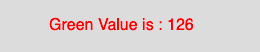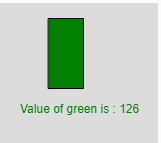# p5.js green() function

The green() function in p5.js is used to extract the green value from a color or pixel array.

Syntax:

`green(c)`

Parameters: This function accepts single parameter c which stores the p5.Color object, color components, or CSS color.

Below programs illustrate the green() function in p5.js:

Example 1: This example uses green() function to extract the green value from a color or pixel array.

 `function` `setup() { ` `     `  `    ``// Create Canvas of size 300*80 ` `    ``createCanvas(300, 80); ` `} ` ` `  `function` `draw() { ` `     `  `    ``// Set background color ` `    ``background(220); ` `     `  `    ``// Initialize the parameter ` `    ``let c = color(0, 126, 255, 102); ` `     `  `    ``// Extract the green value ` `    ``let y = green(c); ` `     `  `    ``// Set font size ` `    ``textSize(16); ` `     `  `    ``// Set font color ` `    ``fill(color(``'red'``)); ` `     `  `    ``// Display result ` `    ``text(``"Green Value is : "` `+ y, 50, 30); ` `}  `

Output:Example 2: This example uses green() function to extract green value and fill it to the rectangle.

 `function` `setup() { ` `   `  `    ``// Create Canvas of size 300*80 ` `    ``createCanvas(160, 180); ` `} ` ` `  `function` `draw() { ` `     `  `    ``// Set the background color ` `    ``background(220); ` `     `  `    ``// Initialize the parameter ` `    ``let c = color(0, 126, 100, 34); ` `     `  `    ``// Sets 'value' to 126 ` `    ``let value = green(c);  ` ` `  `    ``// Fill the color ` `    ``fill(0, value, 0); ` `     `  `    ``// Create rectangle ` `    ``rect(50, 15, 35, 70); ` `     `  `    ``// Display result ` `    ``text(``"Value of green is : "` `+ value, 22, 110); ` `} `

Output:Reference: https://p5js.org/reference/#/p5/green

Whether you're preparing for your first job interview or aiming to upskill in this ever-evolving tech landscape, GeeksforGeeks Courses are your key to success. We provide top-quality content at affordable prices, all geared towards accelerating your growth in a time-bound manner. Join the millions we've already empowered, and we're here to do the same for you. Don't miss out - check it out now!

Previous
Next